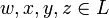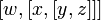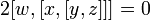# Nilpotency class three is 3-local for Lie rings

This article gives a proof/explanation of the equivalence of multiple definitions for the term Lie ring of nilpotency class three
View a complete list of pages giving proofs of equivalence of definitions

## Statement

The following are equivalent for a Lie ring$L$:

1. The nilpotency class of$L$ is at most three, i.e., we have$[w,[x,[y,z]]] = 0$ for all$w,x,y,z \in L$ (where we allow some or all of$w,x,y,z$ to be equal).
2. The 3-local nilpotency class of$L$ is at most three, i.e., the subring generated by any three elements of$L$ is nilpotent of class at most three.

## Facts used

1. Polarization trick: In particular, this states that alternating implies skew-symmetric.

## Proof

### (1) implies (2)

This is immediate.

### (2) implies (1)

The proof is incomplete.

Given: A Lie ring$L$ of 3-local nilpotency class three

To prove:$[w,[x,[y,z]]] = 0$ for all$w,x,y,z \in L$

Proof:

Step no. Assertion/construction Facts used Given data used Previous steps used Explanation
1 The map$(w,x,y,z) \mapsto [w,[x,[y,z]]]$ is alternating and multi-linear, and hence skew-symmetric, in all pairs of inputs. In particular, this means that its sign is reversed under any odd permutation of the inputs and is preserved under any even permutation of the inputs. Fact (1) 3-local class three For any pair of inputs, if we set them to be equal, then we have a situation of a Lie bracket of length four involving three elements, which must be zero. Hence, we have the alternating condition. Note that the alternating implies skew-symmetric deduction is a special case of Fact (1).
2 For all$w,x,y,z \in L$, we have$3[w,[x,[y,z]]] = 0$. Step (1) By Step (1), we have$[w,[x,[y,z]]] = [w,[y,[z,x]]] = [w,[z,[x,y]]]$. By linearity in$w$ and the Jacobi identity in$x,y,z$, the sum is zero, hence we get the result.
3 For all$w,x,y,z \in L$, we have$[w,[x,[y,z]]] = [[y,z],[w,x]]$ Step (2) Use the Jacobi identity to get$[w,[x,[y,z]]] + [x,[[y,z],w]] + [[y,z],[w,x]] = 0$. Now, the middle term$[x,[[y,z],w]]$ is the negative of$[x,[w,[y,z]]]$, which by the alternating condition we know to be the negative of$[w,[x,[y,z]]]$. So, the middle term equals$[w,[x,[y,z]]]$. Thus, the Jacobi identity expression gives$2[w,[x,[y,z]]] + [[y,z],[w,x]] = 0$. Combine with the conclusion of Step (2) to obtain that$[w,[x,[y,z]]] = [[y,z],[w,x]]$.
4 For all$w,x,y,z \in L$, we have$[y,[z,[w,x]]] = [[w,x],[y,z]]$ Step (3) Interchange the roles of$w$ with$y$, and$x$ with$z$, in Step (3).
5 For all$w,x,y,z \in L$, we have$[[y,z],[w,x]] = [[w,x],[y,z]]$ Steps (1), (3), (4) Combine Steps (3) and (4) with the observation that, by Step (1),$[w,[x,[y,z]]] = [y,[z,[w,x]]]$ because the underlying permutation is even.
6 For all$w,x,y,z \in L$, we have$[[y,z],[w,x]] = -[[w,x],[y,z]]$ Use the skew symmetry of the Lie bracket
7 For all$w,x,y,z \in L$, we have$2[[y,z],[w,x]] = 0$ Steps (5), (6) Step-combination direct
8 For all$w,x,y,z \in L$, we have$2[w,[x,[y,z]]] = 0$ Steps (3), (7) Step-combination direct
9 For all$w,x,y,z \in L$, we have$[w,[x,[y,z]]] = 0$ Steps (2), (8) Step-combination direct